# An Intelligent Control Method for Thermal Inlet of Buildings Based on Thermal Balance of Entire Heat Network

An Intelligent Control Method for Thermal Inlet of Buildings Based on Thermal Balance of Entire Heat Network
Engineering Quality Supervision Center of Logistics Support Department of the Military commission, Beijing 100000, China

Chinese People’s Liberation Army Aviation School, Beijing 100000, China

Corresponding Author Email:
Page:
33-40
|
DOI:
https://doi.org/10.18280/eesrj.100201
21 February 2023
|
Revised:
11 April 2023
|
Accepted:
20 April 2023
|
Available online:
8 May 2023
| Citation

OPEN ACCESS

Abstract:

Heat supply of buildings in reality can be viewed as a process of dynamic changes. Simply increasing the amount of heat supply on the secondary heat network side can result in overheating of near-end users and a greater heat loss. Existing studies generally pay insufficient attention to the pressure fluctuations of heat supply control devices at the thermal inlet of buildings, such as pressure regulating valves and pressure difference balancing valves, the existing methods are of poor applicability to flow-varying systems, so they can not solve the mutual inhibition with the regulating function of temperature control valves at radiator terminals. In view of these matters, this paper studied an intelligent control method for thermal inlet of buildings based on the thermal balance of entire heat network. At first, the structure and principle of heat supply control devices were given; then based on the energy balance method, the heat supply of buildings was adjusted by changing the opening degree of pressure regulating valves on the return pipes, and the specific principle was analyzed in detail. Then, this paper proposed a thermal inlet control method for buildings with the time-based heat supply balance taken into consideration, and constructed a thermal balance equation for heat network based on actual control objectives of operation and regulation. At last, experimental results verified the effectiveness of the proposed method.

Keywords:

thermal balance of heat network, heat supply of buildings, thermal inlet, intelligent control

1. Introduction

The heat supply of buildings in reality can be viewed as a process of dynamic changes. The change of outdoor temperature during heating seasons can cause changes in the heat load of buildings [1-7], however, since often there is no monitoring devices of heat supply at terminals of heat network of buildings, the supply side can only learn the heat supply situation from heat exchange stations, so it can not guarantee that the current heat load of all buildings can match with the heat supply, and the problem of heat supply imbalance occurs sometimes in the heat network, that is, the heat demand of far-end users could not be met from time to time [8-15]. However, simply increasing the amount of heat supply on the secondary heat network side can result in overheating of near-end users and a greater heat loss, so it’s of particular importance to perform scientific operation and regulation on the heat network of buildings from the perspective of the thermal balance of entire heat network [16-19]. Thermal inlet is usually arranged in the trench in front of each building, it consists of valves, pressure regulating orifice plates, pressure gauge, thermometer, and steam trap, etc. By adjusting the heat supply control devices at the thermal inlet of buildings, the heat flow and pressure can be changed to adapt to the operating conditions of buildings so as to meet the residents’ requirement for thermal comfort [20-21].

Tyutyunov et al.  pointed out in their paper that testing and controlling algorithms for temperature conditions in heat supply systems of buildings and structures provide necessary information and support for controlling flows of working media (liquid, gas) in heat load, and such methods enable us to monitor the distribution of heat flows in real time, the authors created a model to control this process and determine the time interval for restarting the heat flow control algorithm in the heat supply system with a dependent connection to the heat source, based on the proposed model, a discretization interval of the heat supply system state probing time was attained, the differential equation of the heat balance of the dependent heat supply system of buildings and structures was studied, and a working formula of discrete interval for controlling the parameters of specified heating system was obtained. Benuzh et al.  introduced the special characteristics of mathematical modeling of process control and argued that in a resource efficient heat supply system of industrial buildings and facilities, constant temperature maintenance is especially critical for the process. In their paper, a functional diagram of operations in the continuous heat supply process was presented, the dependence of temperature at the point of heat-transfer fluid mixing on environmental was analyzed, and a control system operation algorithm was proposed. Jacobs et al.  tell us that the use of Combined Heat Distribution Circuits (CHDC) in apartment buildings is on the rise, authors employed Reinforcement Learning (RL), a machine-learning technique to develop new control strategies for CHDC with floor heating and domestic hot water tanks, and their research findings show that an increasing gamma and decreasing learning rate during training could lead to better performance and that the agent with the largest flexibility had developed a better control strategy that resulted in up to 23% primary energy savings. Sun et al.  discussed a renewable energy technology called the Air Source Heat Pump (AHP) which has been widely used in space heating, during actual application, the setting value of supply water temperature was long-term fixed, resulting in a poor coefficient of performance (COP) under partial load conditions, so in order to improve the COP of ASHP, the authors proposed a real-time variable water temperature (VWT) control method of supply water temperature which calculates optimal supply water temperature based on supply-demand balance and takes it as the setting value of supply water temperature. Then the paper took an ASHP-radiant floor heating system as an example to verify the feasibility and energy saving performance of the proposed control method through simulation experiments.

After reviewing the research of previous scholars in related research fields, it’s found that existing studies generally pay insufficient attention to the pressure fluctuations of heat supply control devices at thermal inlet of buildings, such as pressure regulating valves and pressure difference balancing valves, the existing methods are of poor applicability to variable flow systems, so they can not solve the mutual inhibition with the regulating function of temperature control valves at radiator terminals; in the meantime, existing water supply temperature compensation curves of secondary network are of poor accuracy, and inevitably the response of this temperature adjustment and control compensation method to the heat supply pipe network and enclosure structure has time lag and attenuation features. In view of these matters, this paper studied an intelligent control method for thermal inlet of buildings based on the thermal balance of the entire heat network. In the second chapter, the structure and principle of heat supply control devices were given; then based on the energy balance method, the heat supply of buildings was adjusted by changing the opening degree of pressure regulating valves on return pipes, and the specific principle was analyzed in detail. In the third chapter, this paper proposed a thermal inlet control method for buildings with time-based heat supply balance taken into consideration, and constructed a thermal balance equation for heat network based on actual control objectives of operation and regulation. At last, experimental results verified the effectiveness of the proposed method.

2. Principle of Heat Supply Control Devices

Heat supply control devices are a multi-input multi-output system with time-varying feature, its structure is given in Figure 1. Since heat media in heat pipe network generally have great thermal inertia, if a pipeline is too long, the temperature of return water of the building can not give timely respond to flow changes of heat media, which will result in large inertia and time lag in heat supply control devices. Therefore, in order to make the entire heat network reach the state of thermal balance, two adjustment methods, quality adjustment and quantity adjustment, are usually adopted to adjust the circulation flow of hot water in heat supply network of the building, and the temperature difference control strategy is usually adopted to get a small circulation flow on the basis that the heat load is met.

Assuming: heat load is proportional to indoor-outdoor temperature difference, that is:

$\bar{W}=\bar{H}\left( \frac{{{e}_{h}}-{{e}_{f}}}{{{e}_{h}}^{\prime }-{{e}_{f}}^{\prime }} \right)$       (1)

Quality adjustment method and quantity adjustment method were respectively used to adjust the return water temperature and the hot water circulation flow of heat supply control devices. The formulas below respectively calculate the circulation flow and return water temperature corresponding to the quantity adjustment method:

$\bar{H}=\frac{0.5\left( {{E}_{h}}^{\prime }-{{E}_{f}}^{\prime } \right)\left( \frac{{{E}_{m}}-{{E}_{q}}}{{{E}_{m}}^{\prime }-{{E}_{q}}^{\prime }} \right)}{{{E}_{h}}-{{E}_{m}}-0.5\left( {{E}_{h}}^{\prime }+{{E}_{f}}^{\prime }-2{{E}_{m}} \right)\left( \frac{{{E}_{m}}-{{E}_{q}}^{{1}/{\left( 1+Y \right)}\;}}{{{E}_{m}}^{\prime }-{{E}_{q}}^{\prime }} \right)}$      (2)

${{E}_{f}}=2{{E}_{m}}-{{E}_{h}}^{\prime }+\left( {{E}_{h}}^{\prime }+{{E}_{f}}^{\prime }-2{{E}_{m}} \right){{\left( \frac{{{E}_{m}}-{{E}_{q}}}{{{E}_{m}}^{\prime }-{{E}_{q}}^{\prime }} \right)}^{{1}/{\left( 1+Y \right)}\;}}$       (3)

Between the two adjustment methods, the quantity method has more advantage in terms of energy saving. For direct-connected heat supply network without mixed water, this paper adopted the quantity adjustment method to control the heat supply operation of buildings by changing the opening degree of pressure regulating valves on the return pipe based on energy balance method. Here, the energy balance method sets the control quantity as the amount of heat supplied by the heat network to the building, and the control quantity is the difference between it and the amount of heat actually dissipated from radiator terminals in the building. The quantity adjustment method controls the circulation flow of hot water in the heat supply network of buildings by adjusting the opening degree of pressure regulating valves on the return water pipe in heat supply control devices.

Assuming: W represents the amount of heat supply at thermal inlet, κo represents the specific heat capacity of water, H represents the circulation flow of the pipeline, U represents the external volume of the building, σ represents the density of hot water in the pipeline, Eh represents the water supply temperature at thermal inlet, Ef represents the return water temperature at thermal inlet, then the formula below calculates the amount of heat supply at thermal inlet W:

$W={{\kappa }_{o}}H\left( {{E}_{h}}-{{E}_{f}} \right)=\frac{{{\kappa }_{o}}\sigma U\left( {{E}_{h}}-{{E}_{f}} \right)}{3600}$       (4)

Assuming: Wi represents the amount of heat dissipated from radiator terminals, L represents the heat transfer coefficient of radiator terminals, X represents the heat dissipation area of radiator terminals, Ei represents average indoor temperature, Eoj represents average temperature of heat media in radiator terminals, then the amount of heat actually dissipated from radiator terminals in the building can be calculated by the following formula:

${{W}_{i}}=LX\left( \frac{{{E}_{h}}-{{E}_{f}}}{2}-{{E}_{i}} \right)=LX\left( {{E}_{oj}}-{{E}_{i}} \right)$      (5)

Default W and Wi are equal, considering the thermal balance relationship of heat network shown in Figure 2, the heat supply of the building in any state satisfies:

${{W}_{i}}=W={{\kappa }_{o}}H\left( {{E}_{h}}-{{E}_{f}} \right)=LX\left( \frac{{{E}_{h}}-{{E}_{f}}}{2}-{{E}_{i}} \right)$       (6)

Assuming: Em represents the control quantity of the indoor temperature of the building, then the amount of heat dissipated from radiator terminals is the amount of heat corresponding to Em, that is:

${{W}_{m}}=LX\left( \frac{{{E}_{h}}-{{E}_{f}}}{2}-{{E}_{m}} \right)$      (7)

To maintain thermal balance in the heat network, the indoor temperature of the building should always be kept at Em, then the amount of heat needs to be provided by heat supply control devices is W=Wm=LX(Eoj-Em), and the amount of heat actually provided by heat supply control devices is W=Wi=LX(Eoj-Em). Therefore, if Wi is equal to Wm, then under the condition of a same return water temperature, Ei is equal to Em. If Ei is not equal to Em, then it’s considered that there’s a deviation between Ei and Em, and the heat supply control devices need to adjust the amount of heat supply.

Assuming: x and y represent experimental coefficients, then the formula for calculating heat transfer coefficient of radiator terminals is:

$L=x{{({{E}_{oj}}-{{E}_{i}})}^{b}}=x{{\left( \frac{{{E}_{h}}-{{E}_{f}}}{2}-{{E}_{i}} \right)}^{y}}$       (8)

Assuming: △E=(Eoj-Ei) represents the average temperature difference between heat media in radiator terminals and the indoor air, this temperature difference is an important factor affecting the heat transfer coefficient of radiator terminals and the amount of dissipated heat; assuming β and γ are heat dissipation constants of radiator terminals, by introducing the known E, Formula 3 can be re-written as:

\begin{align} {{W}_{i}}=W={{\kappa }_{o}}H\left( {{E}_{h}}-{{E}_{f}} \right)=LX\left( \frac{{{E}_{h}}-{{E}_{f}}}{2}-{{E}_{i}} \right) =xX{{\left( {{E}_{oj}}-{{E}_{i}} \right)}^{\left( I+y \right)}}=\beta {{\left( {{E}_{oj}}-{{E}_{i}} \right)}^{\gamma }} \end{align}       (9)

Similarly, under the condition that Em is determined, Formula 4 can be re-written as:

\begin{align} {{W}_{m}}=LX\left( \frac{{{E}_{h}}-{{E}_{f}}}{2}-{{E}_{m}} \right)=xX{{\left( {{E}_{oj}}-{{E}_{m}} \right)}^{\left( I+y \right)}}=\beta {{\left( {{E}_{oj}}-{{E}_{m}} \right)}^{\gamma }} \end{align}      (10)

When Wi is greater than WmWi/Wm is greater than 1, that is:

$\frac{{{W}_{i}}}{{{W}_{n}}}=\frac{\beta {{\left( {{E}_{oj}}-{{E}_{i}} \right)}^{\gamma }}}{\beta {{\left( {{E}_{oj}}-{{E}_{m}} \right)}^{\gamma }}}={{\left( \frac{{{E}_{oj}}-{{E}_{i}}}{{{E}_{oj}}-{{E}_{m}}} \right)}^{\gamma }}>1$       (11)

Then it’s solved that Ei is smaller than En. Similarly, when Wi is less than WmWi/Wm is less than 1, then it’s solved that Ei is greater than Em.

According to analysis, if the relationship between Wi and Wm is known, then the relationship between Ei and Em can be judged directly, that is, when Wi=Wm, Ei is equal to Em; when Wi is greater than Wm, Ei is smaller than Em; When Wi is smaller than Wm, Ei is greater than Em.

Then it’s known that, the setting of Em is reflected by Wm, which belongs to the method of controlling the set value, and the range of Ei can be determined by the comparison between Wi and Wm. Therefore, when the energy balance method is adopted to control the heat supply control devices of the building, we’ll only need to judge the real-time relationship between Wi and Wm.

## 1.png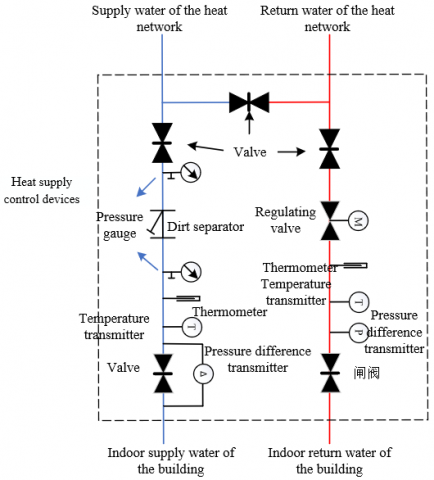Figure 1. Structure of heat supply control devices

## 2.pngFigure 2. Thermal balance relationship of heat network

3. Thermal Inlet Control of Buildings Considering Time-Based Heat Supply Balance

Based on the principle of heat supply control devices, this paper proposed a thermal inlet control method of buildings considering time-based heat supply balance, which not only can supply heat according to demand and meet thermal comfort requirements of users with different heating time requirements, but also solve the thermal imbalance of heat network caused by pipeline network expansion, different radiator terminal conditions, and natural acting pressure head according to the heating characteristics of buildings while saving the energy source to the greatest extent.

By the adopting the said scheme, the indoor temperature demand of buildings during normal operating hours could be met by the heat supply network, while the indoor temperature of buildings during abnormal operating hours could be controlled by adjusting the heating time of heat supply network, that is, appropriately reducing the heating time of over-heating users and increasing the heating time of over-cooling users. By lowering the demand of pipeline network for circulation flow, the scheme can perform uniform heating and on-demand heating of different users, in this way, it could realize accurate heating and maintaining thermal balance in the heat network.

The operation adjustment of heat supply control devices considering time-based heat supply is actually to monitor and measure the temperature and flow of hot water in the heat supply network, the amount of heat gained by radiator terminals, and the system energy consumption using the heat supply control devices according to the heat demand of users and the current indoor and outdoor temperature, and then adjust the amount of heat supply of current users’ requirements by remotely controlling the valve switching frequency. Figure 3 shows the control methods of supplied water and return water at thermal inlet.

Satisfying the indoor temperature demand of users is actually the goal of operation adjustment of heat supply control devices considering time-based heat supply, based on this goal, a basic thermal balance equation could be established for the heat network. Assuming: W1 represents the actual heat supply load of the building, w represents the volume heating index of the building, U represents the external volume of the building, em represents the calculated indoor temperature, eq represents the calculated outdoor temperature, then the heat supply load of the building can be expressed as:

${{W}_{1}}=lG\left( {{e}_{m}}-{{e}_{q}} \right)=wU\left( {{e}_{m}}-{{e}_{q}} \right)$      (12)

Assuming: W2 represents the amount of air-side heat dissipation of radiator terminals, X and Y represent coefficients of radiator terminals determined by experiment, eh represents the predicted water supply temperature, ef represents the predicted water return temperature, Gr represents the design circulation mass flow, then the amount of air-side heat dissipation of radiator terminals is given by the following formula:

${{W}_{2}}=XG{{\left( \frac{{{e}_{h}}+{{e}_{f}}}{2}-{{e}_{m}} \right)}^{Y+1}}=X{{G}_{r}}{{\left( {{e}_{o}}-{{e}_{m}} \right)}^{Y+1}}$      (13)

Assuming: W3 represents the amount of water-side heat dissipation of radiator terminals, H represents the operation circulation mass flow, κ represents the mass specific heat of hot water, then the amount of water-side heat dissipation of radiator terminals is given by the following formula:

${{W}_{3}}=H\kappa \left( {{e}_{h}}-{{e}_{f}} \right)$      (14)

During the normal operating hours of the building, if the heat loss of heat supply network during the process of hot water supply is ignored, the heat supply of the network can be considered as equal to the heat load of the building (namely the amount of heat dissipation of radiator terminals) by default, then there is:

${{W}_{1}}={{W}_{2}}={{W}_{3}}$      (15)

Assuming: W2e represents the amount of air-side heat dissipation of radiator terminals after the temperature of supplied water has been increased, ehe represents the water supply temperature after the temperature of supplied water has been increased. Under the control strategy of stabilizing the return water temperature and adjusting the temperature difference of supply and return water, we can use the following formula to compare the amount of air-side heat of radiator terminals at this time:

$\frac{{{W}_{2}}}{{{W}_{2e}}}=\frac{XG{{\left( \frac{{{e}_{h}}+{{e}_{f}}}{2}-{{e}_{m}} \right)}^{Y+1}}}{XG{{\left( \frac{{{e}_{he}}+{{e}_{f}}}{2}-{{e}_{m}} \right)}^{Y+1}}}={{\left( \frac{\frac{{{e}_{h}}+{{e}_{f}}}{2}-{{e}_{m}}}{\frac{{{e}_{he}}+{{e}_{f}}}{2}-{{e}_{m}}} \right)}^{Y+1}}$     (16)

Assuming: W3e represents the amount of water-side heat dissipation of radiator terminals after the temperature of supplied water has been increased, then the following formula can be used to compare the  amount of water-side heat of radiator terminals:

$\frac{{{W}_{3}}}{{{W}_{3e}}}=\frac{H\left( {{e}_{h}}-{{e}_{f}} \right)}{{{H}_{e}}\left( {{e}_{he}}-{{e}_{f}} \right)}$      (17)

Assuming: W1r represents the design heat load of the building, W2r represents the amount of air-side heat dissipation of radiator terminals under design operating conditions, W3r represents the amount of water-side heat dissipation of radiator terminals under design operating conditions, then, under the operating condition of design outdoor temperature eqr, there is:

${{W}_{1r}}={{W}_{2r}}={{W}_{3r}}$      (18)

Assuming: eqr represents the design outdoor temperature, then the formula for calculating W1r is:

${{W}_{1r}}=lG\left( {{e}_{m}}-{{e}_{qr}} \right)=wU\left( {{e}_{m}}-{{e}_{qr}} \right)$     (19)

Assuming: efr represents the design return water temperature, eor represents the average design supply-return water temperature, then the formula for calculating W2r is:

${{W}_{2r}}=XG{{\left( \frac{{{e}_{h}}+{{e}_{fr}}}{2}-{{e}_{m}} \right)}^{Y+1}}=X{{G}_{r}}{{\left( {{e}_{or}}-{{e}_{m}} \right)}^{Y+1}}$      (20)

Assuming: ehr represents the design water supply temperature, then the formula for calculating W3r is:

${{W}_{3r}}={{H}_{r}}\kappa \left( {{e}_{hr}}-{{e}_{fr}} \right)$      (21)

Under the operating condition of design outdoor temperature eqr, the ratio of heat load W to the heat load under operating condition eqr can be calculated by the following formula:

$\bar{W}=\frac{{{W}_{1}}}{{{W}_{1r}}}=\frac{{{W}_{2}}}{{{W}_{2r}}}=\frac{{{W}_{3}}}{{{W}_{3r}}}$      (22)

Similarly, the ratio of operation circulation mass flow H to the design flow under operating condition eqr can be calculated by the following formula:

$\bar{H}=\frac{H}{{{H}_{r}}}$      (23)

Considering the thermal comfort of users, default the amount of heat dissipation of radiator terminals is proportional to indoor-outdoor temperature difference, then the basic relationship of the operation and adjustment of heat supply control devices considering time-based heat supply can be expressed as:

$\bar{W}=\frac{{{e}_{m}}-{{e}_{q}}}{{{e}_{m}}-{{e}_{qe}}}={{\left( \frac{\frac{{{e}_{h}}+{{e}_{f}}}{2}-{{e}_{m}}}{\frac{{{e}_{hr}}+{{e}_{fr}}}{2}-{{e}_{m}}} \right)}^{Y+1}}=\bar{H}\frac{{{e}_{h}}-{{e}_{f}}}{{{e}_{hr}}-{{e}_{fr}}}$      (24)

## 3.png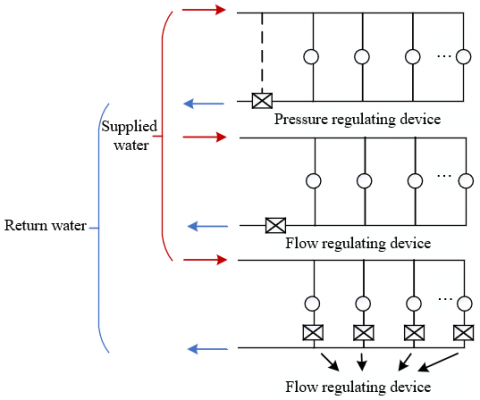Figure 3. Control methods of supplied water and return water at thermal inlet

4. Experimental Results and Analysis

In case that the heat transfer coefficient of radiator terminals was 30%, the time-based heat supply of users didn’t cause much change in the flow at thermal inlet of the building, which had a certain impact on the accuracy of data collection, so in this paper, the heat transfer coefficient of radiator terminals in the method validity verification experiment had been enlarged to make the variation of flow at thermal inlet more obvious. Table 1 shows the influence of time-based heat supply on the total flow at thermal inlet in case of different user numbers. Figure 4 shows the variation of total flow at thermal inlet caused by time-based heat supply in case of different user numbers. The time-based heat supply of users was turned off one by one starting from the bottom layer over-heating users, and the operations of each layer were similar.

Figure 5 and Figure 6 respectively show the relationship between flow control of single user and the total flow and pressure drop at thermal inlet of the building. In this paper, the heat transfer coefficient of radiator terminals was set as 0.5, and the single user flow was controlled at 0, 10, 20, and 30 l/h, respectively. The two column charts give the influence of time-based heat supply of users on the total flow and pressure drop at thermal inlet, the inevitable errors of data collection can result in errors in the thermal inlet of the building, however, judging from the two figures, after adopting the proposed thermal inlet control method considering time-based heat supply balance, the influence of flow control of single user on the total flow and pressure drop of thermal inlet was not much.

Figure 7 and Figure 8 respectively compare the experimental results and model calculation results in case of a 30% and a 50% heat transfer coefficient of radiator terminals. As can be seen from the figure, the experimental results were basically consistent with the calculation results, that is, starting from overheating users in the bottom layer, the heat supply of users was controlled layer by layer, and the comparison clearly showed that, in case that the proposed control method hadn’t been adopted, when the heat transfer coefficient of radiator terminals was 30%, the flow-varying feature at thermal inlet of the building caused by time-based heat supply of users was not obvious, and the increase of the heat transfer coefficient of radiator terminals made the flow-varying feature at thermal inlet become more obvious, but even when the heat transfer coefficient of radiator terminals reached 50%, still the change of total flow at thermal inlet was small, which had further verified that the proposed control method won’t lead to a good flow-varying feature of the thermal inlet of buildings.

Furthermore, this paper analyzed the thermal features of the thermal inlet of buildings, and Table 2 shows the influence of time-based heat supply on other users.

According to Table 2, the feature of constant flow at thermal inlet of the building determined that the influence of time-based heat supply on the flow of other users was small, but there were changes in the amount of heat dissipated from radiator terminals of other users. When time-based heat supply was adopted for bottom layer users, the influence on users in upper layers was small, and when time-based heat supply was adopted for upper layer users, the influence on users in bottom layer was not much as well. In the meantime, compared with constant pressure difference control method, the probability of thermal imbalance in the heat network caused by adopting the constant flow control method at thermal inlet of the building was slightly lower.

To further determine a reasonable time-based heat supply control method, in this paper, the influence of time-based heat supply on flow at thermal inlet under different operating conditions and control methods was compared and analyzed, and the results are given in Figures 9 and 10.

As can be known from the figure, when constant flow control method was adopted at the thermal inlet of buildings, the change in flow caused by time-based heat supply under different operating conditions was small, and there were certain differences in the change of total flow caused by time-based heat supply under vertical and horizontal control methods. In case of a same time-based heat supply ratio of users, whether the position of users is scattered or not determines the degree of influence on the total flow at thermal inlet of buildings. Table 3 gives the flow change caused by time-based heat supply in case that users are extremely scattered. According to the data in the table, the biggest change was close to one-fifth, but in actual cases, it is impossible that all users would choose time-based heat supply or all radiator terminals are completely turned off, so the flow change at thermal inlet of buildings can be ignored. The above results further verified the effectiveness of the proposed method.

Table 1. Influence of time-based heat supply on total flow at thermal inlet of the building in case of different user numbers

 30% Initial 1 3 5 7 9 11 13 Total flow 321 318 318 318 317 302 301 300 Proportion 1 0.994 0.994 0.994 0.99 0.985 0.981 0.974 50% Initial 1 3 5 7 9 11 13 Total flow 324 313 312 308 306 306 300 307 Proportion 1 0.97 0.966 0.958 0.933 0.913 0.885 0.864

Table 2. Influence of time-based heat supply on other users

 Control method Constant flow in stand pipes Constant pressure difference at thermal inlet Layer 3 Layer 2 Layer 1 Layer 3 Layer 2 Layer 1 Heat supply pipeline 1 17.8 19.8 18.1 18 18 18 Layers 1 and 2 are closed / / 25 / / 24.1 Layers 1 and 3 are closed / 21.3 / / 21.3 / Layers 2 and 3 are closed 18 / / 17.9 / / Layer 3 is closed / 21.3 20.9 / 20.8 20.6 Layer 2 is closed 18 / 20.6 18 / 20.6 Layer 1 is closed 18 18 / 18 18 /

Table 3. Flow change caused by time-based heat supply in case that users are extremely scattered

 Control method Control No. Initial condition Pipe 1 Pipes 1 and 2 Pipes 1, 2, and 3 Constant pressure difference at thermal inlet 1 312.6 300.6 304.9 309.6 2 312.9 301.5 307.6 289.6 3 312.5 303.6 283.5 289.6 4 312.4 287.4 283.6 289.6 Constant flow at thermal inlet 1 312.6 350.5 367.6 389.1 2 312.9 352.4 370.9 308.4 3 312.5 354.3 251.6 308.4 4 312.4 285.4 294.6 318.4

## 4.png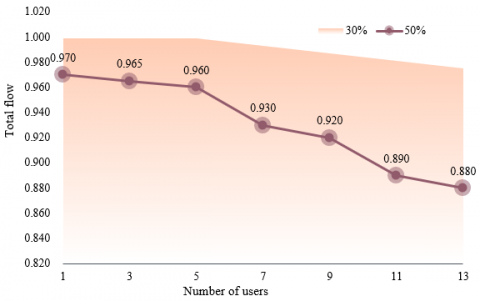Figure 4. Total flow at thermal inlet caused by time-based heat supply in case of different user numbers

## 5.png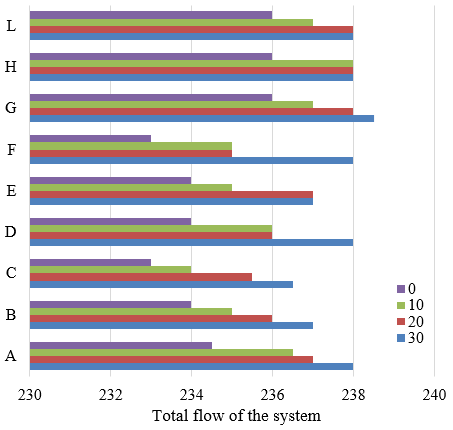Figure 5. Relationship between flow control of single user and the total flow at thermal inlet of the building

## 6.png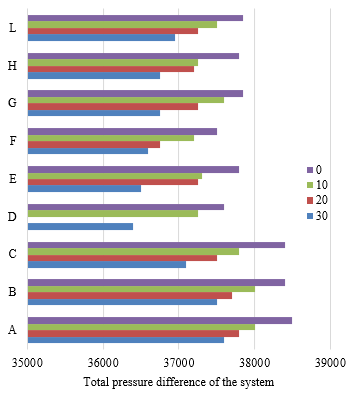Figure 6. Relationship between flow control of single user and pressure drop at thermal inlet of the building

## 7.png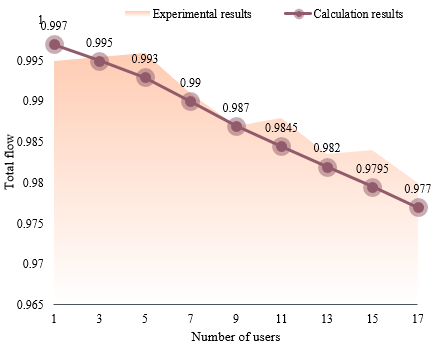Figure 7. Comparison of experimental results and calculation results in case that the heat transfer coefficient of radiator terminals is 30%

## 8.png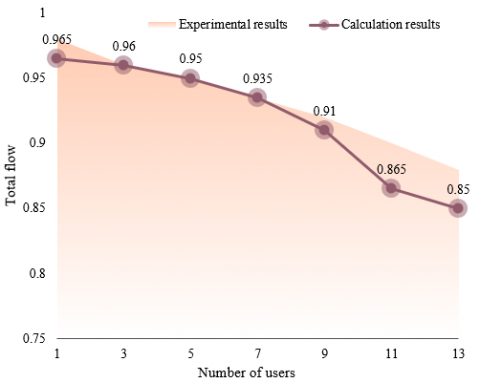Figure 8. Comparison of experimental results and calculation results in case that the heat transfer coefficient of radiator terminals is 50%

## 9.png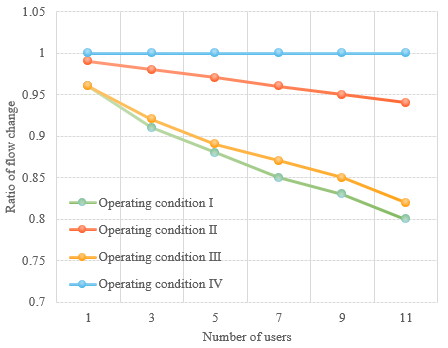Figure 9. Influence of time-based heat supply on flow at thermal inlet

## 10.pngFigure 10. Change of total flow caused by different control methods under constant pressure difference at thermal inlet

5. Conclusion

This paper studied an intelligent thermal inlet control method for buildings based on thermal balance of entire heat network. At first, the structure of heat supply control devices was given, a quantity adjustment method was adopted to control the heat supply operation of the building by changing the opening degree of pressure regulating valves on the return pipe based on energy balance method, and the specific principle was analyzed. Then, this paper proposed a thermal inlet control method of buildings considering time-based heat supply balance, and constructed a thermal balance equation for heat network based on actual control objectives of operation and regulation. The effectiveness of the proposed method was verified by experimental results. In the experiment, the relationship between flow control of single user and the total flow and pressure drop at thermal inlet was analyzed, the experimental results and model calculation results in case of a 30% and a 50% heat transfer coefficient of radiator terminals were compared, which verified that the proposed control method won’t lead to a good flow-varying feature of the thermal inlet of buildings. Moreover, the thermal features of the thermal inlet of buildings were calculated and analyzed, the influence of time-based heat supply on flow at thermal inlet of buildings under different operating conditions and control methods was compared, and the flow change caused by time-based heat supply in case of extremely scattered users was given, which had verified the effectiveness of the proposed method.

References

 Li, T., Wang, Z., Wang, J., Gao, X. (2023). Dynamic performance of organic rankine cycle driven by fluctuant industrial waste heat for building power supply. Energies, 16(2): 1-24. https://doi.org/10.3390/en16020765

 Falk, P.M., Dammel, F., Stephan, P. (2017). Exergy analyses of heat supply systems for a building cluster with CARNOT. International Journal of Thermodynamics (IJoT), 20(4): 191-198. https://doi.org/10.5541/ijot.299926

 Shapoval, S., Venhryn, I., Shapoval, P., Paraniak, N. (2022). Characteristics of a solar collector combined with a building glass facade under different operating modes of the heat carrier in the solar heat supply system. In Proceedings of EcoComfort 2022. Blikharskyy, Switzerland, 2022. Cham: Springer International Publishing. pp. 378-387. https://doi.org/10.1007/978-3-031-14141-6_38

 Abbaspour, A., Yousefi, H., Aslani, A., Noorollahi, Y. (2022). Economic and environmental analysis of incorporating geothermal district heating system combined with radiant floor heating for building heat supply in Sarein, Iran using building information modeling (BIM). Energies, 15(23): 8914. https://doi.org/10.3390/en15238914

 Wang, H., Zhou, P., Guo, C., Tang, X., Xue, Y., Huang, C. (2019). On the calculation of heat migration in thermally stratified environment of large space building with sidewall nozzle air-supply. Building and Environment, 147: 221-230. https://doi.org/10.1016/j.buildenv.2018.10.003

 Guo, X., Goumba, A.P. (2018). Air source heat pump for domestic hot water supply: Performance comparison between individual and building scale installations. Energy, 164: 794-802. https://doi.org/10.1016/j.energy.2018.09.065

 Sandvall, A.F., Ahlgren, E.O., Ekvall, T. (2017). Cost-efficiency of urban heating strategies–Modelling scale effects of low-energy building heat supply. Energy strategy reviews, 18: 212-223. https://doi.org/10.1016/j.esr.2017.10.003

 Tong, J., Quan, Z., Zhao, Y., Wang, G., Cai, J., Chi, Y. (2017). The study on the performance of solar photovoltaic and air source heat pump composite building energy supply system. Procedia Engineering, 205: 4082-4089. https://doi.org/10.1016/j.proeng.2017.09.900

 Wang, Y., Quan, Z., Zhao, Y., Wang, L., Liu, Z. (2022). Performance and optimization of a novel solar-air source heat pump building energy supply system with energy storage. Applied Energy, 324: 119706. https://doi.org/10.1016/j.apenergy.2022.119706

 Pishkar, I. (2022). Using rooftop solar heating to supply part of a high-rise residential building heat in the cold climate of Iran: One-Year dynamic analysis. International Transactions on Electrical Energy Systems, Article ID: 9982264. https://doi.org/10.1155/2022/9982264

 De la Cruz‐Loredo, I., Ugalde‐Loo, C.E., Abeysekera, M. (2022). Dynamic simulation and control of the heat supply system of a civic building with thermal energy storage units. IET Generation, Transmission & Distribution, 16(14): 2864-2877. https://doi.org/10.1049/gtd2.12453

 Jacobson, M.Z. (2021). On the correlation between building heat demand and wind energy supply and how it helps to avoid blackouts. Smart Energy, 1: 100009. https://doi.org/10.1016/j.segy.2021.100009

 Wang, Y., Quan, Z., Jing, H., Wang, L., Zhao, Y. (2021). Performance and operation strategy optimization of a new dual-source building energy supply system with heat pumps and energy storage. Energy Conversion and Management, 239: 114204. https://doi.org/10.1016/j.enconman.2021.114204

 Yang, X., Wang, H., Su, C., Wang, X., Wang, Y. (2020). Heat transfer between occupied and unoccupied zone in large space building with floor-level side wall air-supply system. In Building Simulation, 13: 1221-1233. https://doi.org/10.1007/s12273-020-0639-9

 Pintér, K., Nagy, Z. (2022). Building a UAV based system to acquire high spatial resolution thermal imagery for energy balance modelling. Sensors, 22(9): 3251. https://doi.org/10.3390/s22093251

 Ruiz-Llaneza, N., Alonso-Montolio, C., Isalgue, A., Coch, H. (2020). Heat flux balance in mediterranean climates: Thermal insulation location in building enclosures. In Sustainability in Energy and Buildings , 2020, Littlewood, J., Howlett, R.J., & Jain, L.C. (Eds). Singapore: Springer Singapore, pp. 491-501. https://doi.org/10.1007/978-981-15-8783-2_41

 Kini, P.G., Garg, N.K., Kamath, K. (2017). To investigate the influence of building envelope and natural ventilation on thermal heat balance in office buildings in warm and humid climate. In IOP conference series: earth and environmental science (EES), IOP Publishing, 73(1): 012031. https://doi.org/10.1088/1755-1315/73/1/012031

 Evola, G., Marletta, L., Avola, F. (2020). Energy savings and summer thermal comfort for retrofitted buildings: A complex balance. In Sustainability in Energy and Buildings: Proceedings of SEB 2019. Budapest, Hungary, 2019, Littlewood, J., Howlett, R., Capozzoli, A., & Jain, L. (Eds) Springer Singapore, pp. 281-293. https://doi.org/10.1007/978-981-32-9868-2_24

 Srisamranrungruang, T., Hiyama, K. (2020). Balancing of natural ventilation, daylight, thermal effect for a building with double-skin perforated facade (DSPF). Energy and Buildings, 210: 109765. https://doi.org/10.1016/j.enbuild.2020.109765

 Šteffek, L., Kalánek, J., Ostrý, M. (2014). Analysis and quantification of the influence of thermal properties of building envelope on the energy balance. In Advanced Materials Research. 1041: 146-149. https://doi.org/10.4028/www.scientific.net/AMR.1041.146

 Ballarini, I., Corrado, V. (2012). Analysis of the building energy balance to investigate the effect of thermal insulation in summer conditions. Energy and Buildings, 52: 168-180. https://doi.org/10.1016/j.enbuild.2012.06.004

 Tyutyunov, D., Pihtin, A., Borodin, A. (2022). The Temperature Control Methods for the Heat Supply System of Buildings and Structures. In Modern Problems in Construction: Selected Papers from MPC 2021. Kursk, Russia, 2021, Vatin, N., Pakhomova, E.G., & Kukaras, D. (Eds). Cham: Springer International Publishing, pp. 165-176. https://doi.org/10.1007/978-3-031-12703-8_17

 Benuzh, A., Fedorov, S., Orenburova, E. (2018). Energy efficiency and ecological quality of buildings by process control of heat supply systems. In MATEC Web of Conferences. Moscow, Russia, November 14-16, 2018. EDP Sciences. 251: p.03052. https://doi.org/10.1051/matecconf/201825103052

 Jacobs, S., Ghane, S., Anwar, A., Mercelis, S., Hellinckx, P., Verhaert, I. (2022). Reinforcement learning based mass flow and supply temperature control for combined heat distribution. In IECON 2022-48th Annual Conference of the IEEE Industrial Electronics Society, pp. 1-6.

 Sun, Y., Li, X., Wei, W., Xue, H., Wang, W., Deng, S. (2022). Development of a variable water temperature control method for air source heat pump based on the supply–demand balance. Sustainable Energy Technologies and Assessments, 52: 102366. https://doi.org/10.1016/j.seta.2022.102366